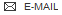BDBM50133220 CHEMBL3634920

SMILES NCCCC[C@H](NC(=O)[C@@H]1CCCN1C(=O)[C@H](CCCNC(N)=N)NC(=O)C1CNC(=O)C[C@@H](NC(=O)[C@H](Cc2ccccc2)NC(=O)CNC(=O)CNC(=O)[C@H](Cc2ccc(O)cc2)NCC2CC2)C(=O)N[C@@H](CCCNC(N)=N)C(=O)N[C@@H](CCCNC(N)=N)C(=O)N1)C(N)=O

InChI Key InChIKey=CKVCQIQNFCFSBW-KWBGLVKESA-N

Data  2 KI

Find this compound or compounds like it in BindingDB:
 Substructure Similarity at least:  must be >=0.5 Exact match

#### Activity Spreadsheet -- Enzyme Inhibition Constant Data from BindingDBFound 2 hits for monomerid = 50133220

TargetKappa-type opioid receptor(Rattus norvegicus (rat))
The University of Kansas

Curated by ChEMBL
Affinity DataKi:  44nMAssay Description:Displacement of [3H]diprenorphine from rat kappa-opioid receptor expressed in CHO cells after 90 mins by scintillation counterMore data for this Ligand-Target Pair
TargetMu-type opioid receptor(Rattus norvegicus (rat))
The University of Kansas

Curated by ChEMBL
Affinity DataKi:  1.09E+4nMAssay Description:Displacement of [3H]DAMGO from rat mu-opioid receptor expressed in CHO cells after 90 mins by scintillation counterMore data for this Ligand-Target Pair